# How to convert decimal number to gray code

## Decimal Number

Whole number, a decimal point and a fractional value combines to form a decimal number. The decimal point separates the whole number part from the fractional part of the number. Each digit of a decimal number can be any number from 0 to 9. Any value less than 1 is written to the right of decimal point. Decimal numbers are also known as base-10 number or counting numbers. Place value of decimal number varies as the whole number powers of 10 starting from the left of decimal point. Similarly, the place value of digits left to decimal point varies as the division of power of tens.

## Gray code

Gray code, also known as reflected binary code, is a code having digits 0 and 1. Gray code do not have place value for its digits. Any successive codes in Gray code system have only one bit changes.

## How to convert decimal to gray code

Decimal number cannot be directly converted to Gray code. Hence, to convert decimal to gray code, first, convert the decimal number to binary. Then, convert the binary to gray code.

Decimal to gray conversion can be well understood from the following example:

### Convert 2510 to gray code

#### Step 1: Convert 2510 to binary.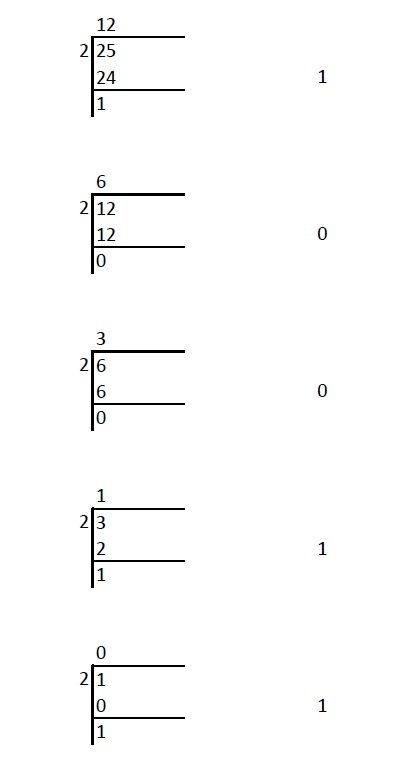Hence, 2510 = 110012

### Read: How to convert decimal to Binary

#### Step 2: Convert 110012 to gray code.

The MSB (Most Significant Bit) of a gray code and binary code will be the same.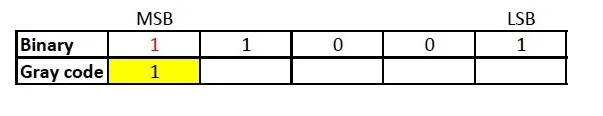The next digit of gray code will be the EXOR of the MSB and the digit right to the MSB of the binary code.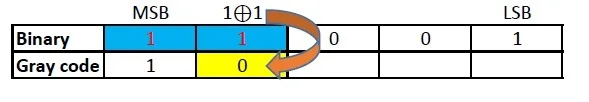Similarly EXOR the digit in place and the previous digit of binary code to obtain the next digit of gray code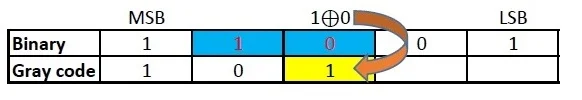Repeat the previous step.Repeat the previous step till the LSB of gray code is found.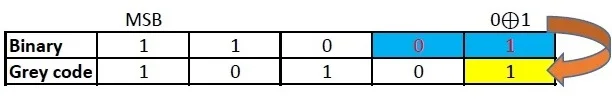Therefore, Gray code corresponding to 2510 = 10101

### This Post Has One Comment

1.George Plousos

hi
I have a simpler method

int(25/02+1/2)=13 odd –> 1
int(25/04+1/2)=06 even –> 0
int(25/08+1/2)=03 odd –> 1
int(25/16+1/2)=02 even –> 0
int(25/32+1/2)=01 odd –> 1

Gray code 25=10101 (from down to up)